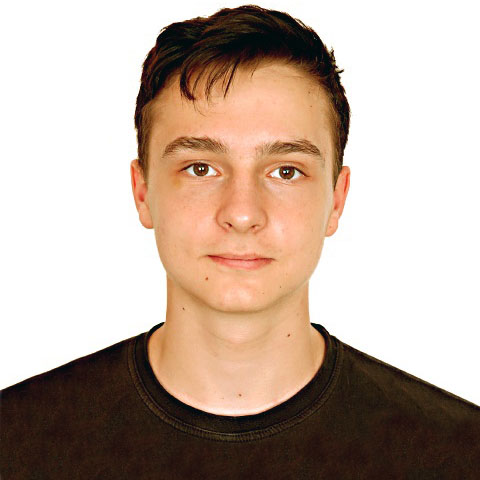e-olymp 8514. Never drink too much!

The task is taken from e-olymp

Mahmoud together with his friends visited Georgia. They would stay in a hotel at Rustavelli. When the cowboys reached the hotel, they hung their hats in the entrance and settled in. The beer bottles on the table could not escape from Mahmoud’s attention when passing through the corridor. At the suggestion of Mahmoud, all the cowboys began drinking. They drank too much, thus none of them was mindful. Then they decided going downtown. On the way out, everyone had a hat on, but they mixed up the hats as they were so drunk.

The man who is able to have on his own hat while he is drunk is considered clever and who is not able to do so is considered stupid.

You are given the number of cowboys — n (including Mahmoud). You should find in how many ways the cowboys may have on the hats so that all of them are stupid. Two ways are considered different if there is at least one cowboy who has a hat in this case and another hat in the other case.

As the answer may become very large, you should output the result modulo 109 + 7.

Input

Given the number of cowboys — n (1 ≤ n ≤ 107).

Output

The answer to the problem as specified above.

Tests

 № Inputs Outputs 1 1 0 2 4 9 3 9 133349 4 555 335132588 5 10000000 824182295

Solution

We have all possible permutations $C_n^0 \cdot n!$ , minus one fixed (one of them is not stupid) we get $C_n^0 \cdot n!-C_n^1 \cdot (n-1)!$ but we subtract for minimum fixed pair (two of them are not stupid) we need to add them $C_n^0 \cdot n!-C_n^1 \cdot (n-1)! + C_n^2 \cdot (n-2)!$ etc.
So the $k$ member is $C_n^k \cdot (n-k)! \cdot (-1)^k$
Lets find the attitude of $k$ member to the previous one. Its $-k -1$
The last one will be $-1$ it depends on parity of $n$.
First two are the same ( $n!$ ) so we skip them, but in this case we need to check if$n$ equals $1$
And next we make a loop to find the answer by multiplying start value and add it to the answer.
The complexity is $O(n)$

3 thoughts on “e-olymp 8514. Never drink too much!”

1.Мазурок Игорь Евгеньевич says:
• Пожалуйста, используйте TeX для формул в условии.
• В чем смысл использованных макроопределений?
• Не стоит использовать return вместо else.
•Руслан Масальский says:

Спасибо, исправил

2.Олег Петров says:

Поддержу Игоря Евгеньевича. Переопределять int — очень нехорошая практика. В результате даже пришлось определять main() как int32_t. В большом проекте за такие дефайны сотрудники точно не поблагодарят. Если Вам лень писать long long или Вы предполагаете дальнейшее переопределние какого-то типа данных, можете использовать незарезервированный псевдоним. И безопаснее его оформлять через typedef, а не #define. Например:
typedef long long ll;